# Image-to-Image Translation using Pix2Pix

• Last Updated : 27 Jan, 2022

pix2pix was proposed by researchers at UC Berkeley in 2017. It uses conditional Generative Adversarial Network to perform the image-to-image translation task (i.e. converting one image to another, such facades to buildings and Google Maps to Google Earth, etc.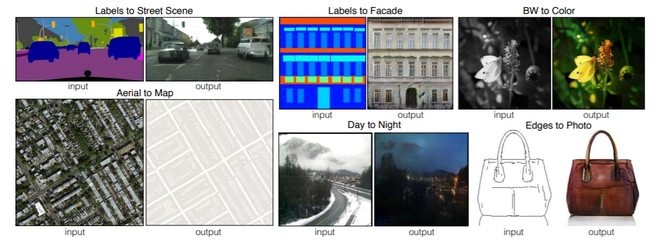## Architecture :

The pix2pix uses conditional generative adversarial networks (conditional-GAN) in its architecture. The reason for that is that even if we trained a model with a simple L1/L2 loss function for a particular image-to-image translation task, this might not understand the nuances of the images.

Generator: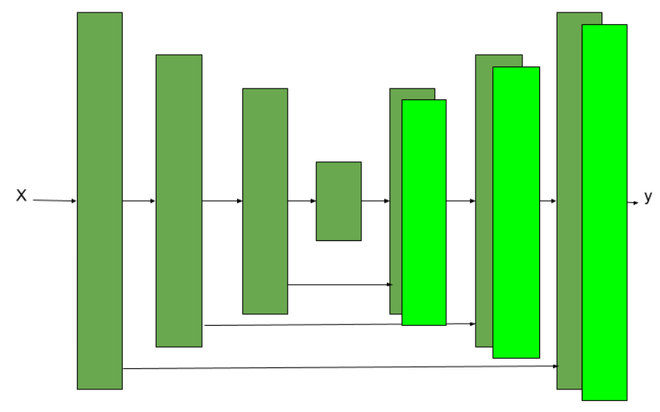U-Net architecture

The architecture used in the generator was U-Net architecture. It is similar to Encoder-Decoder architecture except for the use of skip-connections in the encoder-decoder architecture. The use of skip-connection makes this

• Encoder Architecture: The Encoder network of the Generator network has seven convolutional blocks. Each convolutional block has a convolutional layer, followed by a LeakyRelu activation function (with a slope of 0.2 in the paper). Each convolutional block also has a batch normalization layer except the first convolutional layer.
• Decoder Architecture: The Decoder network of the Generator network has seven Transpose convolutional block. Each upsampling convolutional block (Dconv) has an upsampling layer, followed by a convolutional layer, a batch normalization layer, and a ReLU activation function.
• The generator architecture contains skip connections between each layer i and layer n − i, where n is the total number of layers. Each skip connection simply concatenates all channels at layer i with those at layer n − i.

Discriminator:

The discriminator use Patch GAN architecture, which is also used Style GAN architecture. This PatchGAN architecture contains a number of Transpose convolutional blocks. This PatchGAN architecture takes an NxN part of the image and tries to find whether it is real and fake. This discriminator is applied convolutionally across the whole image, averaging it to generate the result of the discriminator D.

Each block of the discriminator contains a convolution layer, batch norm layer, and LeakyReLU. This discriminator receives two inputs:

• The input image and Target Image (which discriminator should classify as real)
• The input image and Generated Image (which discriminator should classify as fake).

THe PatchGAN is used because the author argues that it will be able to preserve high-frequency details in the image, with low-frequency details that can be focused by L1-loss.

Generator Loss:

The generator loss used in the paper is the linear combination of L1- loss between generated image, target image, and GAN loss as we define above.Our generated loss will be:Therefore, our total loss for generatorDiscriminator Loss

The discriminator loss takes two inputs real image and generated image:

• real_loss is a sigmoid cross-entropy loss of the real images and an array of ones(since these are the real images).
• generated_loss is a sigmoid cross-entropy loss of the generated images and an array of zeros(since these are the fake images)
• The total loss is the sum of the real_loss and generated_loss.

Implementation:

• First, we download and preprocess the image dataset. We will use the CMP Facade dataset that was provided Czech Technical University and processed by the authors of the pix2pix paper. We will preprocess the dataset before training.

Code:

## python3

 # import necessary packagesimport tensorflow as tf import osimport time from matplotlib import pyplot as pltfrom IPython import display# install tensorboard ! pip install -U tensorboard # download datasetURL = "https://people.eecs.berkeley.edu/~tinghuiz / projects / pix2pix / datasets / facades.tar.gz" path_to_zip = tf.keras.utils.get_file('facades.tar.gz',                                      origin = URL,                                      extract = True) PATH = os.path.join(os.path.dirname(path_to_zip), 'facades/') # Define Training variableBUFFER_SIZE = 400BATCH_SIZE = 1IMG_WIDTH = 256IMG_HEIGHT = 256 # load the images from datasetdef load(image_file):  image = tf.io.read_file(image_file)  image = tf.image.decode_jpeg(image)   w = tf.shape(image)   w = w // 2  real_image = image[:, :w, :]  input_image = image[:, w:, :]   input_image = tf.cast(input_image, tf.float32)  real_image = tf.cast(real_image, tf.float32)  return input_image, real_image # resize the images to provided width and heightdef resize(input_image, real_image, height, width):  input_image = tf.image.resize(input_image, [height, width],                                method = tf.image.ResizeMethod.NEAREST_NEIGHBOR)  real_image = tf.image.resize(real_image, [height, width],                               method = tf.image.ResizeMethod.NEAREST_NEIGHBOR)   return input_image, real_image """function to stack (input, real) images and apply random crop on them to cropto (256, 256)"""def random_crop(input_image, real_image):  stacked_image = tf.stack([input_image, real_image], axis = 0)  cropped_image = tf.image.random_crop(      stacked_image, size =[2, IMG_HEIGHT, IMG_WIDTH, 3])   return cropped_image, cropped_image """Before training, we need to perform random jittering on the datasetAccording to the paper, this random jittering contains 3 steps --> Resize the image to bigger size --> Random crop the image to target size of model --> Random Flip on the images """ @tf.function()def random_jitter(input_image, real_image):  # resizing to 286 x 286 x 3  input_image, real_image = resize(input_image, real_image, 286, 286)   # randomly cropping to 256 x 256 x 3  input_image, real_image = random_crop(input_image, real_image)   if tf.random.uniform(()) > 0.5:    # random mirroring    input_image = tf.image.flip_left_right(input_image)    real_image = tf.image.flip_left_right(real_image)   return input_image, real_image
• Now, we load train and test data using the function we defined above.

Code:

## python3

 # function to Load image from train data"""On train data, we performed random jitter and normalize,but since we don't need any augmentation on test_data, we just resize it"""def load_image_train(image_file):  input_image, real_image = load(image_file)  input_image, real_image = random_jitter(input_image, real_image)  input_image, real_image = normalize(input_image, real_image)   return input_image, real_image# function to Load images from test datadef load_image_test(image_file):  input_image, real_image = load(image_file)  input_image, real_image = resize(input_image, real_image,                                   IMG_HEIGHT, IMG_WIDTH)  input_image, real_image = normalize(input_image, real_image)   return input_image, real_image # apply the above load_images_train function on train datatrain_dataset = tf.data.Dataset.list_files(PATH+'train/*.jpg')train_dataset = train_dataset.map(load_image_train,                                  num_parallel_calls = tf.data.experimental.AUTOTUNE)train_dataset = train_dataset.shuffle(BUFFER_SIZE)train_dataset = train_dataset.batch(BATCH_SIZE) # apply the above load_images_test function on test datatest_dataset = tf.data.Dataset.list_files(PATH+'test/*.jpg')test_dataset = test_dataset.map(load_image_test)test_dataset = test_dataset.batch(BATCH_SIZE)
• After performing data processing, Now, we write the code for generator architecture. This generator block contains 2 parts encoder block and decoder block. The encoder block contains a downsampling convolution block and the decoder block contains an upsampling transpose convolution block.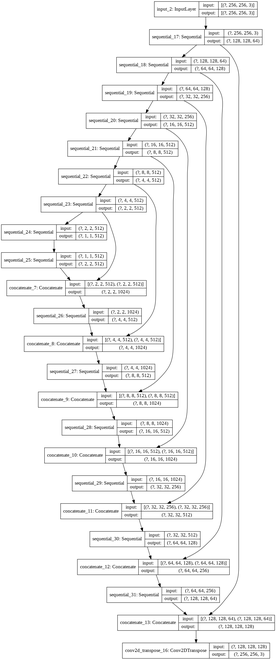Generator architecture

• Now we define our architecture for the discriminator. The discriminator architecture uses a PatchGAN model. For this architecture, we can use the above downsampling convolution block we defined. The loss of the discriminator is the sum of real loss (sigmoid cross-entropy b/w real image and array of 1s) and generated loss (sigmoid cross-entropy b/w generated image and an array of 0s).

Code:

## python3

 # code for discriminator architecture"""FOr more details look into architecture section"""def Discriminator():  initializer = tf.random_normal_initializer(0., 0.02)   inp = tf.keras.layers.Input(shape =[256, 256, 3], name ='input_image')  tar = tf.keras.layers.Input(shape =[256, 256, 3], name ='target_image')   x = tf.keras.layers.concatenate([inp, tar]) # (batch_size, 256, 256, channels * 2)   down1 = downsample(64, 4, False)(x) # (batch_size, 128, 128, 64)  down2 = downsample(128, 4)(down1) # (batch_size, 64, 64, 128)  down3 = downsample(256, 4)(down2) # (batch_size, 32, 32, 256)   zero_pad1 = tf.keras.layers.ZeroPadding2D()(down3) # (batch_size, 34, 34, 256)  conv = tf.keras.layers.Conv2D(512, 4, strides = 1,                                kernel_initializer = initializer,                                use_bias = False)(zero_pad1) # (batch_size, 31, 31, 512)   batchnorm1 = tf.keras.layers.BatchNormalization()(conv)   leaky_relu = tf.keras.layers.LeakyReLU()(batchnorm1)   zero_pad2 = tf.keras.layers.ZeroPadding2D()(leaky_relu) # (batch_size, 33, 33, 512)   last = tf.keras.layers.Conv2D(1, 4, strides = 1,                                kernel_initializer = initializer)(zero_pad2) # (batch_size, 30, 30, 1)   return tf.keras.Model(inputs =[inp, tar], outputs = last) # define discriminator loss functiondisc_ce_loss = tf.keras.losses.BinaryCrossentropy(from_logits = True)def discriminator_loss(disc_real_output, disc_generated_output):  real_loss = disc_ce_loss(tf.ones_like(disc_real_output), disc_real_output)   generated_loss = disc_ce_loss(tf.zeros_like(disc_generated_output), disc_generated_output)   total_disc_loss = real_loss + generated_loss   return total_disc_loss discriminator = Discriminator()tf.keras.utils.plot_model(discriminator, show_shapes = True)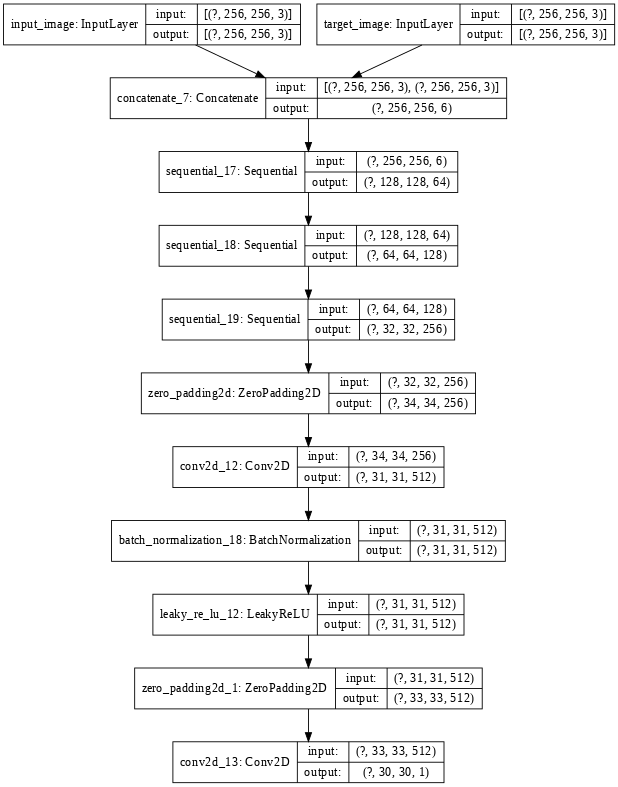Discriminator Architecture

• In this step, we define optimizers and checkpoints. We will use Adam optimizer in both generator discriminator.

Code:

## python3

 # define generator and discriminator architecturegenerator_optimizer = tf.keras.optimizers.Adam(2e-4, beta_1 = 0.5)discriminator_optimizer = tf.keras.optimizers.Adam(2e-4, beta_1 = 0.5) # Create the model checkpointcheckpoint_dir = './train_checkpoints'checkpoint_prefix = os.path.join(checkpoint_dir, "ckpt")checkpoint = tf.train.Checkpoint(generator_optimizer = generator_optimizer,                                 discriminator_optimizer = discriminator_optimizer,                                 generator = generator,                                 discriminator = discriminator)
• Now, we define the training procedure. The training procedure consists of the following steps:
• For each example input, we passed the image as input to the generator to get the generated image.
• The discriminator receives the input_image and the generated image as the first input. The second input is the input_image and the target_image.
• Next, we calculate the generator and the discriminator loss.
• Then, we calculate the gradients of loss with respect to both the generator and the discriminator variables(inputs) and apply those to the optimizer.

Code:

## python3

 # Define training procedureEPOCHS = 30 import datetimelog_dir ="logs/" summary_writer = tf.summary.create_file_writer(  log_dir + "fit/" + datetime.datetime.now().strftime("% Y % m % d-% H % M % S")) @tf.functiondef train_step(input_image, target, epoch):  with tf.GradientTape() as gen_tape, tf.GradientTape() as disc_tape:    gen_output = generator(input_image, training = True)     disc_real_output = discriminator([input_image, target], training = True)    disc_generated_output = discriminator([input_image, gen_output], training = True)     gen_total_loss, gen_gan_loss, gen_l1_loss = generator_loss(disc_generated_output, gen_output, target)    disc_loss = discriminator_loss(disc_real_output, disc_generated_output)   generator_gradients = gen_tape.gradient(gen_total_loss,                                          generator.trainable_variables)  discriminator_gradients = disc_tape.gradient(disc_loss,                                               discriminator.trainable_variables)   generator_optimizer.apply_gradients(zip(generator_gradients,                                          generator.trainable_variables))  discriminator_optimizer.apply_gradients(zip(discriminator_gradients,                                              discriminator.trainable_variables))   with summary_writer.as_default():    tf.summary.scalar('gen_total_loss', gen_total_loss, step = epoch)    tf.summary.scalar('gen_gan_loss', gen_gan_loss, step = epoch)    tf.summary.scalar('gen_l1_loss', gen_l1_loss, step = epoch)    tf.summary.scalar('disc_loss', disc_loss, step = epoch)     def fit(train_ds, epochs, test_ds):  for epoch in range(epochs):    % time     for example_input, example_target in test_ds.take(1):      generate_images(generator, example_input, example_target)    print("Epoch: ", epoch)    # Train    for n, (input_image, target) in train_ds.enumerate():             train_step(input_image, target, epoch)    print()    # saving (checkpoint) the model every 10 epochs    if (epoch + 1) % 10 == 0:      checkpoint.save(file_prefix = checkpoint_prefix)  checkpoint.save(file_prefix = checkpoint_prefix)fit(train_dataset, EPOCHS, test_dataset)
• Now, we use the generator of the trained model on test data to generate the images.

Code:

## python3

 # code to plot resultsdef generate_images(model, test_input, tar):  prediction = model(test_input, training = True)  plt.figure(figsize =(15, 15))   display_list = [test_input, tar, prediction]  title = ['Input Image', 'Ground Truth', 'Predicted Image']   for i in range(3):    plt.subplot(1, 3, i + 1)    plt.title(title[i])    # getting the pixel values between [0, 1] to plot it.    plt.imshow(display_list[i] * 0.5 + 0.5)    plt.axis('off')  plt.show()   for inputs, tar in test_dataset.take(5):  generate_images(generator, inputs, tar)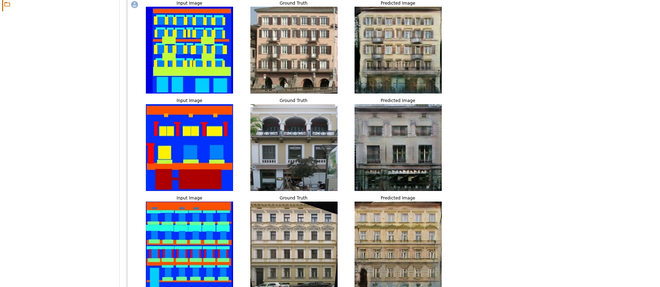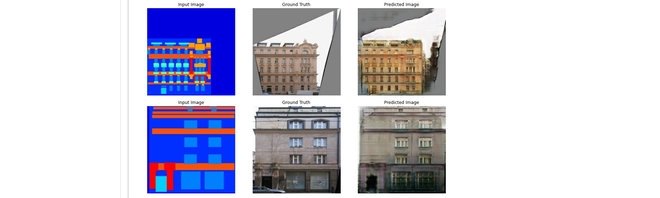Results

References:

My Personal Notes arrow_drop_up